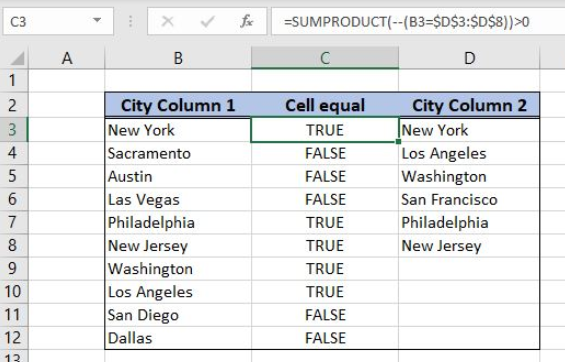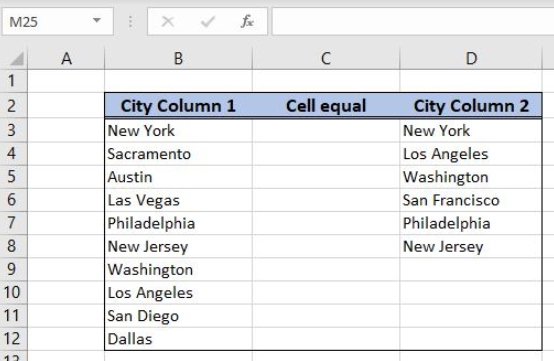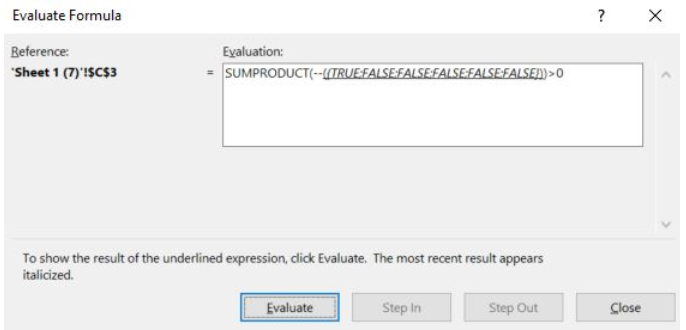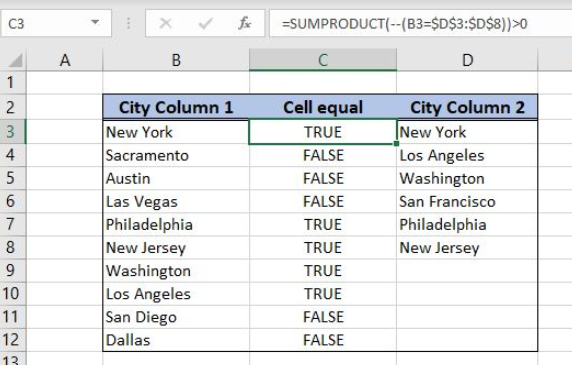Get instant live expert help with Excel or Google Sheets“My Excelchat expert helped me in less than 20 minutes, saving me what would have been 5 hours of work!”

#### Post your problem and you’ll get Expert help in seconds.

Your message must be at least 40 characters
Our professional Expert are available now. Your privacy is guaranteed.

# Cell equals one of many things

Excel allows a user to check if a cell is equal to one or many things from a range, using the SUMPRODUCT function. As a result, we get True or False. This step by step tutorial will assist all levels of Excel users in checking if a cell is equal to one or many values from another range.Figure 1. The result of the SUMPRODUCT function

## Syntax of the SUMPRODUCT Formula

The generic formula for the SUMPRODUCT function is:

`=SUMPRODUCT(--(cell = array))`

The parameters of the SUMPRODUCT function are:

• cell – a cell which we want to find in an array
• array – an array where we look up for a cell.

The function compares cell with cells from an array one by one and returns Booleans TRUE or FALSE. Using the double negation “–” in front of the logical test, the Booleans are converted to 1 or 0. In the end, the function sums everything and returns a result.

## Setting up Our Data for the SUMPRODUCT FunctionFigure 2. Data that we will use in the SUMPRODUCT example

In columns B and C we have two lists of cities which we want to compare. In the cell F2, we want to return the total number of matches between two columns.

## Using the SUMPRODUCT to Count Matches Between Two Columns

In our example, we want to check if cells from column A (“City column 1”) is equal to any city in column C (“City column 2”). If it is, the value in column B will be True, otherwise, it will be False.

The formula looks like:

`=SUMPRODUCT(--(B3 = \$D\$3:\$D\$8)) > 0`

The cell is B3 and array is \$D\$3:\$D\$8. We need to fix the array, as we are always looking in this array.

To apply the SUMPRODUCT function, we need to follow these steps:

• Select cell C3 and click on it
• Insert the formula: `=SUMPRODUCT(--(B3 = \$D\$3:\$D\$8)) > 0`
• Press enter
• Drag the formula down to the other cells in the column by clicking and dragging the little “+” icon at the bottom-right of the cell.

Let’s evaluate the formula and see the result:Figure 3. Evaluate the SUMPRODUCT function

As you can see, the function has TRUE if the cell B3 is found in the array D3:D8 or FALSE if not. The first value from D3:D8 array is equal to B3, so we have TRUE for this value. These values are converted to 1 and 0 and summed. The sum is 1 which is greater than 0, so the result in C3 is True.Figure 4. Using the SUMPRODUCT to check if the cell equals one of many things

Most of the time, the problem you will need to solve will be more complex than a simple application of a formula or function. If you want to save hours of research and frustration, try our live Excelchat service! Our Excel Experts are available 24/7 to answer any Excel question you may have. We guarantee a connection within 30 seconds and a customized solution within 20 minutes.

### Did this post not answer your question? Get a solution from connecting with the expert.Another blog reader asked this question today on Excelchat:
Solution examplesUse the Vlookup Function to complete the "employee" column of table 2. Use "job Id" from table 2 as your lookup_value(s) and table 1 as your reference.
Solved by C. H. in 16 minsIf a cell in another sheet is populated I need a vlookup done. If the cell is not populated I need the cell to return blank.
Solved by T. D. in 60 minsI am trying to make a chart that turns a week range red if nothing is entered in the range. If something is entered then I would like it to turn green. Please Help
Solved by E. U. in 43 minsI need a check box to show/hide an answer of an if function
Solved by Z. U. in 23 minsI need a formula to compare the data in two columns and then export the mismatched data in the 3rd column
Solved by S. Q. in 20 mins## Subscribe to Excelchat.coAnother blog reader asked this question today on Excelchat: